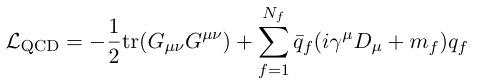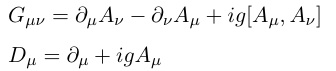## Dr. Y. Koma's Research Topics

[ ]
 Topics

• Quantum chromodynamics
• It is well-known that particles like protons and neutrons, which are generally called "hadrons", are basic ingredient of nuclei. The size of a hadron is quite small such that its typical diameter is about 1/1000 000 000 000 000 meter (= 10^{-15} m = 1 fm). Surprisingly, hadrons are not yet "fundamental" particles and there exist further smaller particles inside them called "quarks" and "gluons".

So far, various collision experiments of hadrons using accelerators strongly suggest that the dynamics of quarks and gluons are described by the theory called "quantum chromodynamics (QCD)". QCD is a quantum gauge field theory associated with SU(3) group symmetry, and its lagrangian density is given bywheredenote the field strength tensor and covariant derivative. "q" and "A" represent the quark and the gluon fields, respectively. The coupling "g" controls the strength of the interaction of quarks and gluons which depends on the energy scale of QCD to be applied.

However, it is not straightforward to solve QCD due to its nonperturbative nature, and there are still unsolved problems even after the discovery in the 1970s. For instance, one never observe an isolated quark so far in the accelerator experiment, which means that quarks are somehow confined inside hadrons. This is called "quark confinement" problem.

Studying nonperturbative nature of QCD, using lattice QCD simulations and effective low-energy field theories of QCD, is my current research theme.

 Keywords

• Lattice QCD simulations
• Define QCD in Euclidean space in four dimensions on a hypercubic discrete lattice and compute the QCD partition function by the Monte-Carlo method using supercomputers.